# Transitioning from counting to multiplying to find area | 3rd grade | Khan Academy

##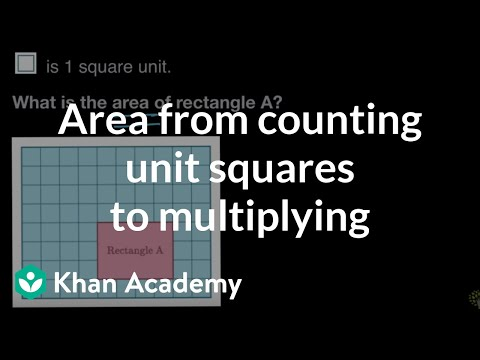By Khan Academy

finds the area of a rectangle both by counting unit squares and multiplying side lengths# Multiplying decimals 3

##By Khan Academy

Sometimes multiplying really small decimals (with all those zeros!) can be a little intimidating. Watch as we show you a handy trick to simplify these problems and solve them.# Multiplying decimals 3

##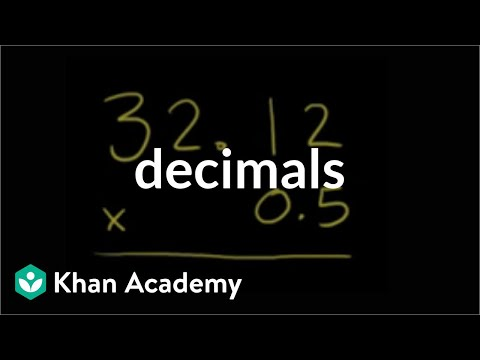By Khan Academy

Multiplying decimals? Try multiplying without the decimals first, them add them back in. We'll show you.# 6.RP.3 - Solve Word Problems Using Tape Diagrams

##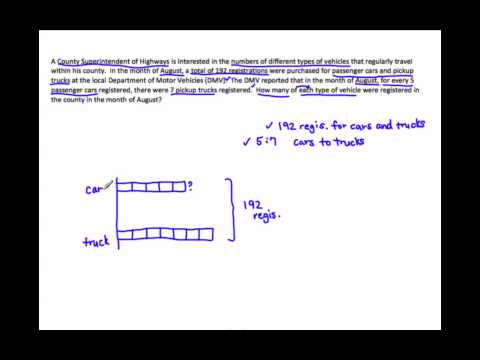By MathwithMrAlmeida

In this video, Mr. Almeida shows you how to solve ratio word problems using tape diagrams. The models create equivalent ratios because you are multiplying both quantities in one ratio by the same positive number (the length of 1 unit). This meets Common Core Standard for Mathematics 6.RP.3.# More involved multiplying decimals example

##By Khan Academy

Sometimes multiplying really small decimals (with all those zeros!) can be a little intimidating. Watch as we show you a handy trick to simplify these problems and solve them.# Multiplying fractions by fractions word problems

##By Khan Academy

Someone is about to run out of laundry detergent which means sticky clothes! Help her estimate how much she has left.# Multiplying fractions by fractions word problems

##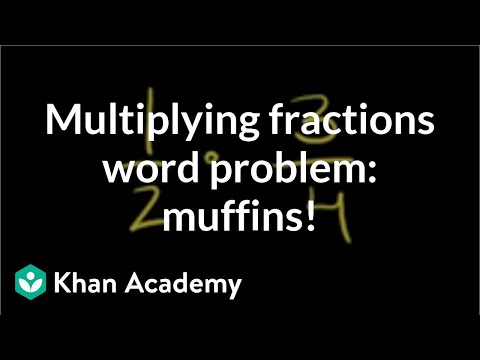By Khan Academy

We hope you learn to cook. Everyone should! At some point you'll need to make 1/2 a recipe which means using 1/2 of every ingredient in the recipe. Here's an example.# Multiplying fractions by fractions word problems

##By Khan Academy

You know how long it takes to bike to your friend's house, and you know how fast your can ride. So how far away does your friend live? The answer is one fraction multiplication problem away!# Multiplying and Dividing Decimals | 7.NS.A.2c | 7th Grade Math

##By ParksMath | Todd Parks

ParksMath explains how to multiply and divide decimals numbers without the use of a calculator. Understanding how to change a decimal number so that it is an integer, makes multiplying and dividing decimals much easier. This quick tutorial will give you thee tools that you need to find the product or quotient of any decimal number.# Algebra 1: Section 12.4- Long Division of Polynomials

##By Megan Frantz

Long Division of Polynomials# Adding and Subtracting Polynomials (Simplifying Math)

##By Eric Buffington

A short lesson on adding and subtracting Polynomials. This video emphasizes the importance of adding and subtracting like terms.# Multiplying Mixed Numbers - YourTeacher.com - Pre Algebra Help

##By yourteachermathhelp

For a complete lesson on multiplying mixed numbers go to http://www.yourteacher.com - 1000+ online math lessons featuring a personal math teacher inside every lesson! In this lesson students learn to multiply mixed numbers by first rewriting the mixed numbers as improper fractions then multiplying the improper fractions together then rewriting the resulting improper fraction as a mixed number if necessary. For example to multiply 1 8/15 x 1 1/4 first rewrite the mixed numbers as improper fractions to get 23/15 x 5/4. Next cross-cancel the 15 and 5 to 3 and 1 and we have 23/3 x 1/4. Next multiply across the numerators and denominators to get 23/12. Finally rewrite 23/12 as the mixed number 1 11/12.# Multiplying Decimals by Whole Numbers

##By SmithMathAcademy

Multiplying Decimals by Whole Numbers# Divide polynomials by monomials with remainders

##By Khan Academy

Sal divides (18x^4-3x^2+6x-4) by 6x.# Divide polynomials by linear binomials with remainders

##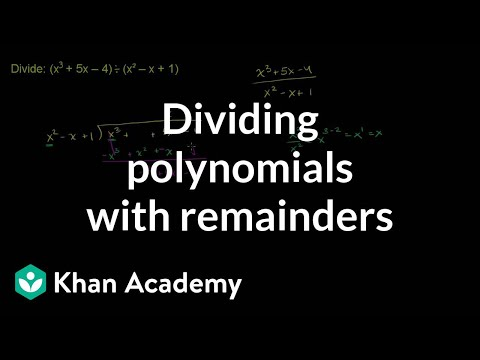By Khan Academy

Sal divides (x^3+5x-4) by (x^2-x+1) using long division.# Divide polynomials by linear binomials with remainders

##By Khan Academy

Sal explains what polynomial long division is, and gives various examples of polynomial long divisions.# Divide polynomials by monomials with remainders

##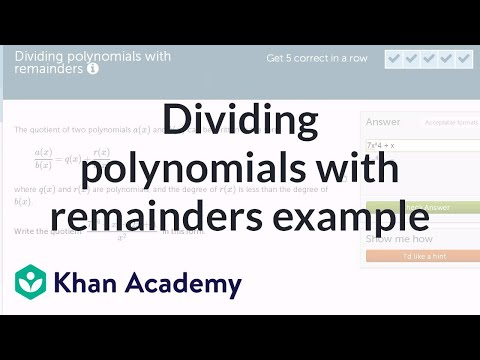By Khan Academy

Sal divides (7x^6+x^3+2x+1) by X^2, and writes the solution as q(x)+r(x)/x^2, where the degree of the remainder, r(x), is less than the degree of x^2.# Finding percents

##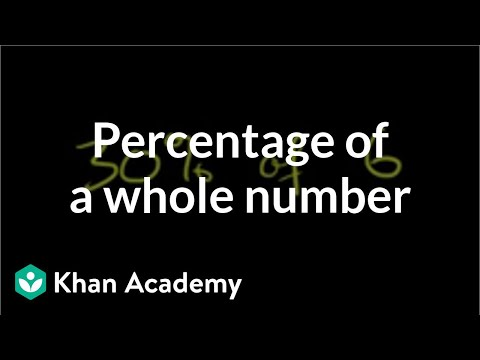By Khan Academy

We hope you're beginning to see that there's more than one way to skin a cat. In other words, there are several different ways to solve problems involving percentages, decimals, and fractions. Watch as find the percentage of a whole number.# Area models to visualize division using place value | 4th grade | Khan Academy

##By Khan Academy

We can break up big division problems into smaller, easier problems. In this video, we solve 268 divided by 2 and 856 divided by 8.# One-step equations with multiplication and division

##By Khan Academy

This equation can be simplified through a single step to solve for the variable. Can you help?